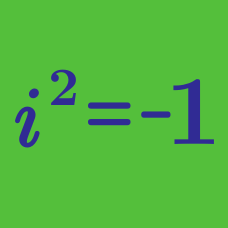Algebra

# Complex Numbers: Level 2 Challenges

What is

$\large i^2 +i^6 +i^4?$

Note: $i$ is the imaginary number $\sqrt{-1}$.

$\displaystyle\sum_{n=0}^{1000} i^n=?$ where $i=\sqrt{-1}$.

$\large i{ z }^{ 3 }+z^{ 2 }-z+i=0$

For $i = \sqrt{-1}$, $z$ is a complex number that satisfies the equation above. What is the value of $\left| z \right|$?

Evaluate

$\large{ \frac { { (1+i) }^{ 85 } }{ { (1-i) }^{ 83 } }}$

Hint: There's an extremely fast way to solve this if you know why
$\large 1+i = \sqrt{2}e^{i{\pi}/{4}}$

Can you evaluate this giant power of $i$?

$\huge i^{-9999}$

×